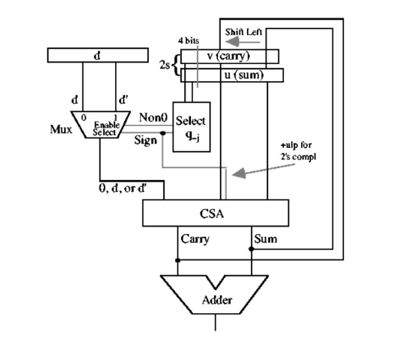# Implementation of a Generic Fixed Point Divider

The goal is to design and implement the HDL of a high-performance hardware serial divider for high frequencies. Initially, at least two different division algorithms will be investigated and analyzed. The design will be parametrized so that it can be configured according to specified requirements. The divider will support a variety of input / output number representation formats.Dividing two numbers to compute the quotient and the remainder sounds very easy, but quite a few academic papers have been written about algorithms for implementing an efficient fixed point divider. The best companies are competing to implement an optimized divider with the highest frequency with the smallest area.

Understanding how HDL code is converted into hardware by the synthesis tool and the use well-known optimized blocks for arithmetic operations such as addition will enable the user to implement an efficient design.

In a system that requires a lot of calculations to be done as fast as possible, for example in a signal processing / image processing system, a good hardware divider can make the difference between success and failure of the whole system.

Project Goal:

The goal is to design and implement the HDL of a high-performance hardware serial divider for high frequencies. Initially, at least two different division algorithms will be investigated and analyzed. The design will be parametrized so that it can be configured according to specified requirements. The divider will support a variety of input / output number representation formats.

Project Requirements:

• Comparison of division algorithms and selection of the best architecture.
• Implementation of a pipelined divider that receives as inputs two numbers (dividend and divisor) and after a determined number of cycles produces the result (quotient and remainder).
• The block interface will be AXI STREAM on and off.
• The divider will be parametrized and can be configured as needed.
• The divider will support different number representation formats: signed, unsigned, fixed point
• The divider will support different calculation accuracy.
• Build a simulation environment and fully test all requirements.
• Synthesis and layout.

Supervisor : Pavel Gushpan : pavel.gushpan@gmail.com